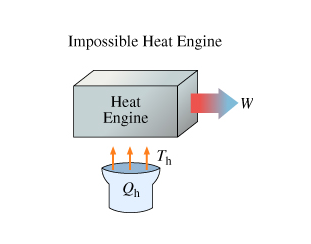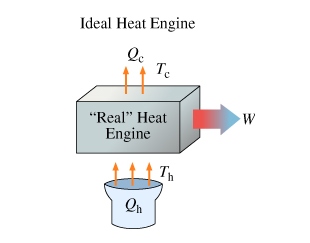# Problem: Learning Goal: To understand what a heat engine is and its theoretical limitations.Ever since Hero demonstrated a crude steam turbine in ancient Greece, humans have dreamed of converting heat into work. If a fire can boil a pot and make the lid jump up and down, why can't heat be made to do useful work?A heat engine is a device designed to convert heat into work. The heat engines we will study will be cyclic: The working substance eventually returns to its original state sometime after having absorbed a quantity of heat and done some work. A cyclic heat engine cannot convert heat into work without generating some waste heat in the process. Although by no means intuitively obvious, this is an important fact of nature, since it dramatically affects the technology of energy generation. If it were possible to convert heat into work without any waste heat, then one would be able to build refrigerators that are more than 100% efficient!Consequently, the "impossible heat engine" pictured schematically here (Intro 1 figure) cannot exist, even in theory. Engineers tried hard for many years to make such a device, but Sadi Carnot proved in 1824 that it was impossible.The next figure (Intro 2 figure) shows an "ideal" heat engine, one that obeys the laws of thermodynamics. It takes in heat Qh at a temperature Th and does work W . In the process of doing this it generates waste heat Qc at a cooler temperature Tc .Take Qh and Qc to be the magnitudes of the heat absorbed and emitted, respectively; therefore both quantities are positive.picture a) picture b)A) Qh&gt;Qc and Th&gt;TcB) Find the work done by the "ideal" heat engine. express W in terms of Qh and QcC) The thermal efficiency of a heat engine is defined as follows: e=W/Qh. express e in terms of Qh and Qc

###### FREE Expert Solution

A.

The conditions that must hold for heat engines to do work are.

Qh>Qc and Th>Tc###### Problem Details

Learning Goal: To understand what a heat engine is and its theoretical limitations.

Ever since Hero demonstrated a crude steam turbine in ancient Greece, humans have dreamed of converting heat into work. If a fire can boil a pot and make the lid jump up and down, why can't heat be made to do useful work?

A heat engine is a device designed to convert heat into work. The heat engines we will study will be cyclic: The working substance eventually returns to its original state sometime after having absorbed a quantity of heat and done some work. A cyclic heat engine cannot convert heat into work without generating some waste heat in the process. Although by no means intuitively obvious, this is an important fact of nature, since it dramatically affects the technology of energy generation. If it were possible to convert heat into work without any waste heat, then one would be able to build refrigerators that are more than 100% efficient!

Consequently, the "impossible heat engine" pictured schematically here (Intro 1 figure) cannot exist, even in theory. Engineers tried hard for many years to make such a device, but Sadi Carnot proved in 1824 that it was impossible.

The next figure (Intro 2 figure) shows an "ideal" heat engine, one that obeys the laws of thermodynamics. It takes in heat Qh at a temperature Th and does work W . In the process of doing this it generates waste heat Qc at a cooler temperature Tc .

Take Qh and Qc to be the magnitudes of the heat absorbed and emitted, respectively; therefore both quantities are positive.

picture a)picture b)A) Qh>Qc and Th>Tc

B) Find the work done by the "ideal" heat engine. express W in terms of Qh and Qc

C) The thermal efficiency of a heat engine is defined as follows: e=W/Qh. express e in terms of Qh and Qc